## Example Questions

← Previous 1

### Example Question #1 : How To Find The Part From The Whole

If a TV show is 45 minutes long, what fraction of the show is completed 5 minutes after it begins?

1/4

1/9

1/3

1/2

1/10

1/9

Explanation:

5 minutes out of 45 have been completed. We can represent this as a fraction: 5/45. When simplified, this yields 1/9.

### Example Question #2 : How To Find The Part From The Whole

A birthday cake is cut into 8 pieces.  On the first night three pieces are eaten.  On the second night, 20% of the remaining cake was eaten.  On the third night, half of the remaining cake was eaten.  How many slices were left to eat on the fourth night?

1

3

2

4.5

1/2

2

Explanation:

First night: 8 – 3 = 5 remaining.

Second night: 20% of 5 remaining = .2 * 5 = 1 slice Therefore 4 remaining.

Third night: ½ of the 4 remaining = ½*4 = 2 slices remaining

### Example Question #3 : How To Find The Part From The Whole

There was a malfunction at a candy factory and the machines only put red, purple, and green candy into the bags.  If 1/4 of the candies were red and 3/8 were green, how many purple candies were in the bag, if each bag can hold 16 pieces?

6

8

10

4

3

6

Explanation:

Since 1/4 of the 16 are red: 16 * 1/4 = 4 red candies.  With 3/8 being green:

16 X 3/8 = 6 green candies.   16 – 4 – 6 = 6 candies remain, which must be purple.

### Example Question #1 : Whole And Part

In the 30-day month of January, for every three days it snowed, there were seven days it did not snow. The number of days in January on which it did not snow was how much greater than the number of days in January on which it snowed?

11

14

10

13

12

12

Explanation:

The question tells us that for every ten-day period in January (a three-day period plus a seven-day period), it snowed on 3 of those days and did not snow on 7 of those days. Since January has 30 days, it has 3 ten-day periods, so we multiply the numbers given for the 10-day period by 3 to find the  number of days with and without snow during the 30-day period. Doing this, we see that it snowed 3 * 3 = 9 days and did not snow 7 * 3 = 21 days during the 30-day period. Since the question asks how much greater the number of days on which it did not snow is than the number of days on which it snowed, we subtract as follows: number of days it did not snow - number of days it snowed = 21 – 9 = 12.

### Example Question #5 : How To Find The Part From The Whole

Mikey has one full box of cereal.  On Saturday, he eats 1/3 of the the box.  On Sunday he eats 2/3 of what is left.  How much of the box is still left?

5/9

none

2/3

4/9

2/9

2/9

Explanation:

If Mikey eats 1/3 of the box, he is left with 1 – 1/3 = 2/3.

When he eats 2/3 of what remains, he is eating 2/3 of 2/3, or 4/9 of the box.

2/3 – 4/9 = 2/9

### Example Question #6 : How To Find The Part From The Whole

What is the remainder when 27 is divided by 6?

4

0.5

3

4.5

8

3

Explanation:

Long division is the fastest way: 6 goes into 27 four times. 6 times four is 24. 27 – 24 = 3.

### Example Question #7 : How To Find The Part From The Whole

A pitcher is currentlyof the way full of water.  Ifounces are poured out, then the pitcher would beof the way full.  How many ounces of water are in the pitcher before theounces are poured out?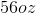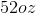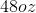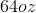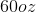Explanation:

Let p represent the total capacity of the pitcher.  The current volume in the pitcher is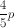.  If this volume is depleted byounces, it becomes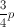.  In other words: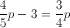Solve this equation for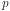and get: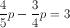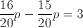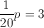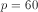Remember,represents the total capacity of the pitcher, or 60 ounces.  The question asks how many ounces are in the pitcher at the beginning of the problem, so evaluate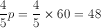.

### Example Question #1 : Whole And Part

Mr. Owens spent $7.50 for a dinner buffet. The amount he paid accounted for 3/4 of the money in his wallet. How much money is left in his wallet for other expenses? Possible Answers:$2.50

$6.50$1.00

$4.00$10.00

$2.50 Explanation: If$7.50 is 3/4 of the total, 7.50/3 gives us what 1/4 of his total money would be. This equals \$2.50, the remaining unspent quarter.

### Example Question #1 : How To Find The Whole From The Part

A certain ball that is dropped will bounce back to 3/5 of the height it was initially dropped from.  If after the 2nd bounce the ball reaches  39.96 ft, what was the initial height the ball was dropped from?

66 ft

135 ft

150 ft

100 ft

111 ft

111 ft

Explanation:

We know the height of the initial bounce, so work backwards to find the initial height.  39.96/0.6 = 66.6 = height of ball after first bounce

66.6/0.6 = 111 ft

### Example Question #3 : Whole And Part

A pitcher of water is filled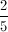of full.  An additional 27 ounces of water is added.  Now the pitcher of water is completely full.  How much water does the pitcher hold?

40

50

45

35

30

If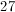ounces fills the pitcher, then it must equal the volume of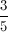of the pitcher.  Ifof a pitcher equals 27 ounces, then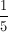of a pitcher equals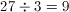ounces.  Since there arefifths in the pitcher, it must hold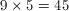ounces total.